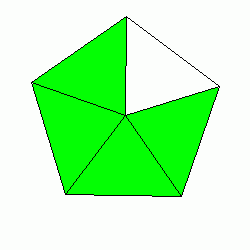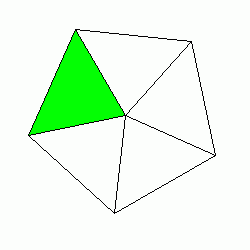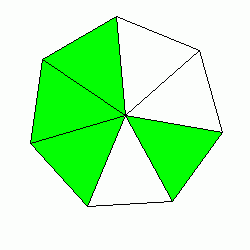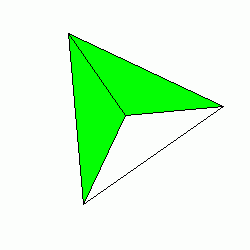# Math Practice Topic: Probability

 Description: This topic covers probability problems. You may need pencil and paper. Adaptive Learning Progression: Numbers get bigger. Start using MathScore for free

## Sample Levels (out of 7)

Find the probability that a randomly selected piece of the shape will be highlighted and find the odds that a piece chosen will not be highlighted. Express probabilities as a simplified fraction and odds as a ratio of two numbers with no common factors other than 1 (i.e. "2:1").

1.Probability: Odds:

2.Probability: Odds:

Find the probability that a randomly selected piece of the shape will be highlighted and find the odds that a piece chosen will not be highlighted. Express probabilities as a simplified fraction and odds as a ratio of two numbers with no common factors other than 1 (i.e. "2:1").

1.Probability: Odds:

2.Probability: Odds: